Static Equilibrium

FxTotal = FyTotal = TorqueNet = 0

Ex#11. Now let’s us examine the question of the hanging sign. The system below is in static equilibrium, find the force, FW, acting on the beam by the wall.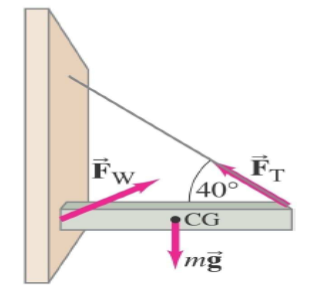Ex#12: Find the tension force, FT, and the force, FH, acting at the hinge of the beam in the diagram shown below. (Please note that the mass of the sign is 34.0 kg and that the mass of the beam is 12.0 kg). [Also please ignore the small distance the sign is hung from the edge of the beam].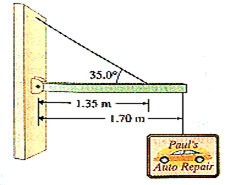Assume Ff(wall) = 0 (unless stated otherwise)

 Situation Physics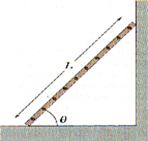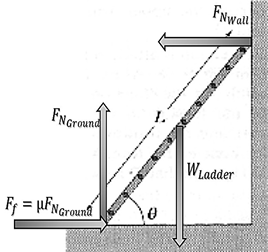Physics Statements: Since      ∑Fx = ∑ Fy = ∑ τ = 0, then: (Wladder)(0.5Lcosθ) = (FNwall)(Lsinθ)      and          FNground = Wladder             FNwall = Ff                                    BUT            Ff  = μFN                     so μ = (FNwall)/(WLadder)

Ex#13. A uniform ladder is L = 5.0 m long and weighs 400.0 N. The ladder rests against a slippery vertical wall, as shown in Figure. The inclination angle between the ladder and the rough floor is θ = 53°. Find the reaction forces from the floor and from the wall on the ladder and the coefficient of static friction μk at the interface of the ladder with the floor that prevents the ladder from slipping.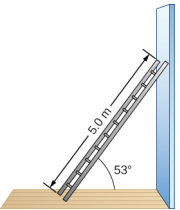Ex#14. Find the coefficient of friction for the system below given that the system is in static equilibrium.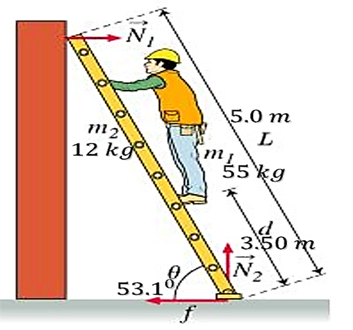Ex#15. Find the tension, T, in the system shown below. The system is in static equilibrium. The mass of the beam is 24.0 kg and its length is 2.4 m.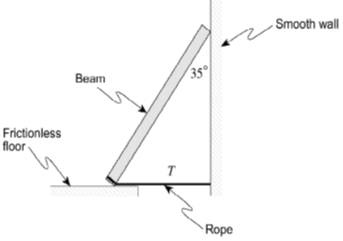Practice1. A beam of negligible mass is attached to a wall by means of a hinge. Attached to the centre of the beam is a 400 N weight. A rope also helps to support this beam as shown in the diagram.
a) What is the tension in the rope?                                                                                                                                                                      (311 N)
b) What are the vertical and horizontal forces that the wall exerts on the beam?                                                                            (V: 200 N, H: 238 N)2. Find the tension in the rope supporting the 200 N hinged uniform beam as shown in the diagram.                                                                                                                                                                                                                                                                                                            (200 N)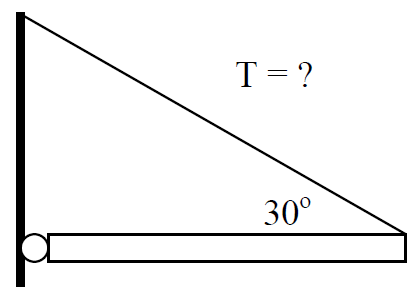3. Find the tension in the rope supporting the 200 N hinged uniform beam as shown in the diagram.                                                                (83.9 N)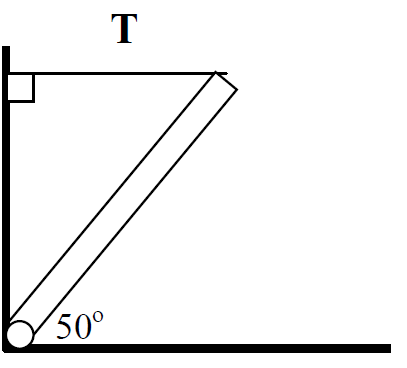4. A uniform beam (mass = 22 kg) is supported by a cable that is attached to the centre of the beam a shown in the diagram.
a) find the tension in the cable.                                                                                                                                                                          (1000 N)
b) find the horizontal and vertical forces acting on the hinge.                                                                                                 (Fx = 710 N, Fy = 240 N)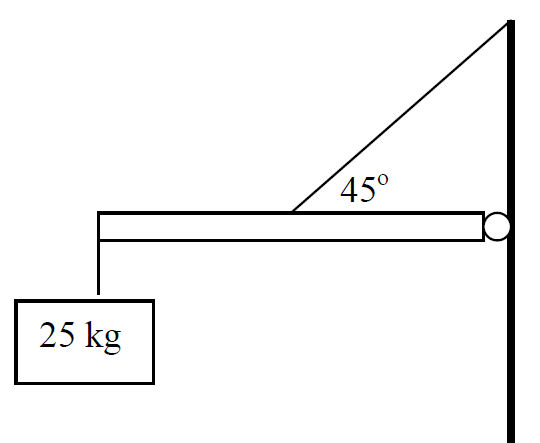5. The diagram below shows the top view of a door that is 2 m wide. Two forces are applied to the door as indicated in the diagram. What is the net torque on the door with respect to the hinge?                                                                                                                                   (8.66 Nm clockwise)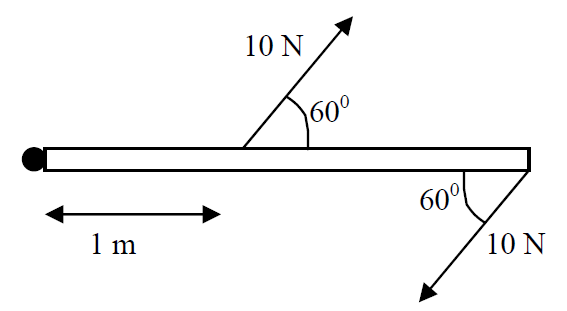6. A 2.6 m uniform beam (mass of 9.0 kg) is attached to a wall by a hinge and supported by a rope. A 13 kg mass hangs from the beam 2.2 m from the hinge. Find the tension in the rope which is attached to the beam 1.1 m from the wall.                                                                                  (770 N)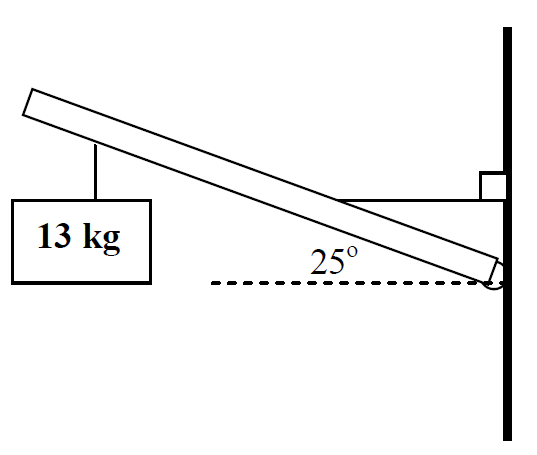7. A uniform ladder of mass 12.5 kg leans against a wall at an angle of 730 to the horizontal. The coefficient of static friction between the ladder and the wall and between the ladder and the ground is 0.76. How heavy of a person can climb all the way to the top of the ladder before the ladder starts to slip?

8. A box of uniform density weighing 100 newtons moves in a straight line with constant speed along a horizontal surface. The coefficient of sliding friction is 0.4 and a rope exerts a force F in the direction of motion as shown below.

a. On the diagram above, draw and identify all the forces on the box.
b. Calculate the force F exerted by the rope that keeps the box moving with constant speed.
C. A horizontal force F', applied at a height 5/3 meters above the surface as shown in the diagram above, is just sufficient to cause the box to begin to tip
forward about an axis through point P. The box is 1 meter wide and 2 meters high. Calculate the force F’.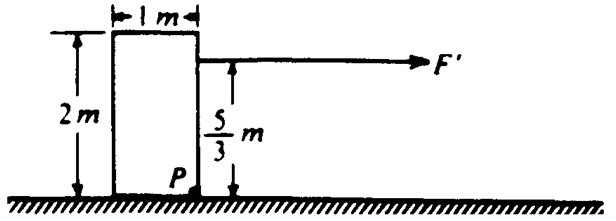D

9. A long, uniform rod of mass M and length l is supported at the left end by a horizontal axis into the page and perpendicular to the rod, as shown above. The right end is connected to the ceiling by a thin vertical thread so that the rod is horizontal. Express the answers to all parts of this question in terms of M, l and g.
A. Determine the magnitude and direction of the force exerted on the rod by the axis.
B. If the breaking strength of the thread is 2Mg, determine the maximum distance, r, measured from the hinge axis, that a box of mass 4M could be placed without breaking the thread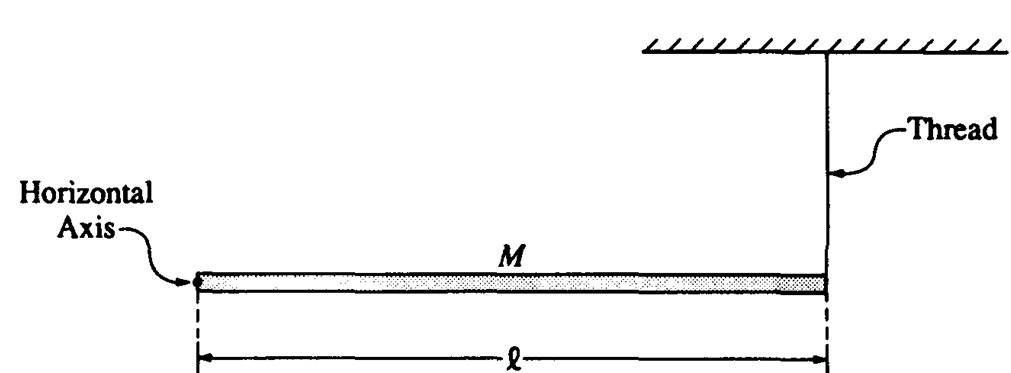10. As shown above, a uniform disk is mounted to an axle and is free to rotate without friction. A thin uniform rod is rigidly attached to the disk. A block is attached to the end of the rod. Properties of the rod, and block are as follows.
Rod: mass = m, length = 2R
Block: mass = 2m
The system is held in equilibrium with the rod at an angle θ to the vertical, as shown above, by a horizontal string of negligible mass with one end attached to the disk and the other to a wall. Determine the tension in the string in terms of m, θ, and g.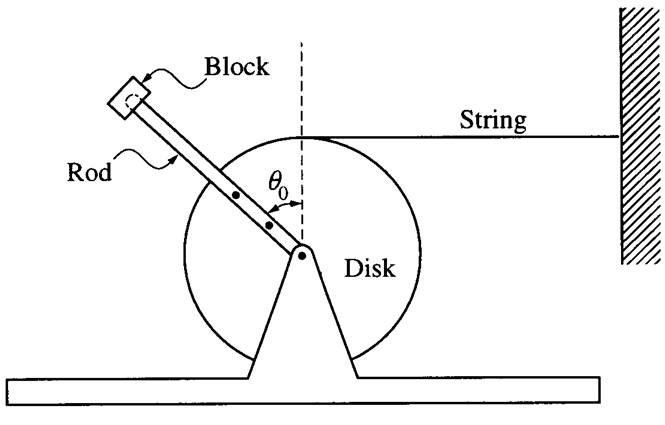11. Two masses. m1 and m2, are connected by light cables to the perimeters of two cylinders of radii r1 and r2, respectively, as shown in the diagram above with r1 = 0.5 meter, r2 = 1.5 meters, and m1 = 20 kilograms. Determine m2 such that the system will remain in equilibrium.12. The horizontal uniform rod shown above has length 0.60 m and mass 2.0 kg. The left end of the rod is attached to a vertical support by a frictionless hinge that allows the rod to swing up or down. The right end of the rod is supported by a cord that makes an angle of 30° with the rod. A spring scale of negligible mass measures the tension in the cord. A 0.50 kg block is also attached to the right end of the rod.
A. On the diagram above, draw and label vectors to represent all the forces acting on the rod. Show each force vector originating at its point of application.
B. Calculate the reading on the spring scale.
C. Calculate the magnitude of the force exerted by the hinge on the rod.

13. The diagram below shows a beam of length 20.0 m and mass 40.0 kg resting on two supports placed at 5.0 m from each end. A person of mass 50.0 kg stands on the beam between the supports. The reaction forces at the supports are shown.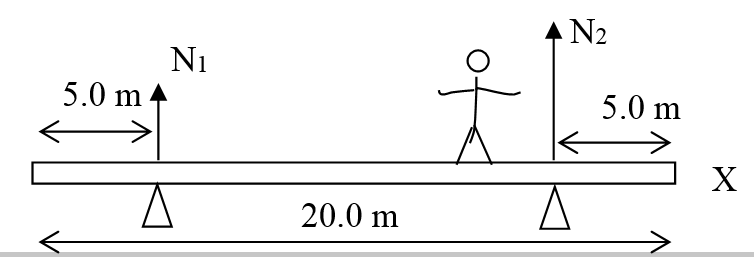(a) State the value of N1 + N2
(b) The person now moves toward the X end of the beam to the position where the beam just begins to tip and reaction force N1 becomes zero as the beam starts to leave the left support. Determine the distance of the girl from the end X when the beam is about to tip.